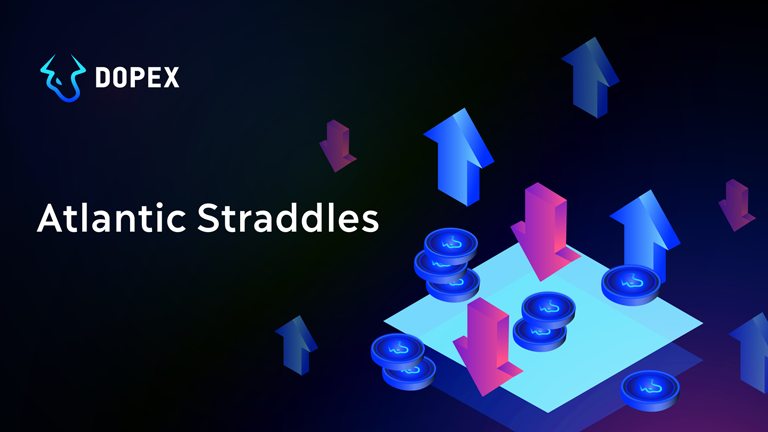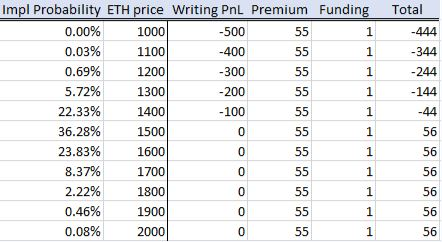# Atlantic Strategies: Providing Liquidity for Atlantic Straddles

Dopex-EssentialsDopex-Papers# HOW DOES IT WORK?

###### During this phase the USDC collateral will be used to sell the Atlantic Puts to the buyers. You may be surprised - Atlantic Puts? Not a mix of Puts and Calls? Yes - the Puts only. Recall the formula we introduced above and let us go through the following example:
• The buyer places an order to buy a straddle for a total notional of 1 ETH (0.5 ETH call and 0.5 ETH put) and pays the premium in USDC
• The pool sells 1 ETH put to the buyer
• The half of the collateral is borrowed by the buyer (50% x 1500 USD = 750 USD) and is used to purchase ETH
###### The LP’s position is:
• 1 short Atlantic Put
• Premium from selling the Put
• Interest on the lent out portion
• 1 long Atlantic Put
• 0.5 ETH spot

# LET’S TALK SOME NUMBERS

###### Let us use the following assumptions:
• ETH spot price = 1500 USD
• Time to expiry = 3 days
• Implied volatility = 100%
• Funding rate = 16%
###### With the above parameters, we will see the following premiums:
• Premium for 1 ETH put = 55 USD
• Premium for 1 ETH call = 55 USD
• Premium for 0.5 ETH put = 27.5 USD
• Premium for 0.5 ETH call = 27.5 USD
######If we take a closer look at the above tables, we can notice the following:
• The LPs will collect a steady stream of premiums and fundings every 3 days
• The LPs are subjected to a downside risk